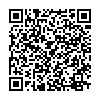## Catalog# 指针和数组

## 指针和一维数组间的关系

### 用下标法引用数组元素

#include<stdio.h>
int main()
{
int a, i;
for(i = 0; i < 5; i++)
scanf("%d", &a[i]);
for(i = 0; i < 5; i++)
printf("%d ", a[i]);
return 0;
}

#include<stdio.h>
int main()
{
int a, i;
for(i = 0; i < 5; i++)
scanf("%d", a + i);    //这里a + i等价于&a[i]
for(i = 0; i < 5; i++)
printf("%d ", *(a + i));    //这里a + i等价于a[i]
return 0;
}

*a 表示取出首地址 a 所指的存储单元中的内容，即元素 a*(a + i) 表示取出首地址元素后第 i 个元素的内容，即下标为 i 的元素 a[i]

### 指针运算的特殊性及其在访问数组元素中的作用

#include<stdio.h>
int main()
{
int a, *p;
for(p = a; p < a + 5; p++)
scanf("%d", p);
for(p = a; p < a + 5; p++)
printf("%d ", *p);
return 0;
}

p + 1p++ 本质上是两个不同的操作，虽然二者都对指针变量 p 进行加1运算，但 p + 1 并不改变当前指针的指向， p 仍然指向原来指向的元素，而 p++ 相当于执行 p = p + 1 ，因此， p++ 操作改变了指针 p 的指向，表示将指针变量 p 向前移动一个元素位置，即指向了下一个元素。

#include<stdio.h>
int main()
{
int  a, *p = NULL, i;
p = a;    //p = a等价于p = &a
for (i = 0; i < 5; i++)
{
scanf("%d", &p[i]);    //&p[i]等价于p + i
}
p = a;    //在再次循环开始前，确保指针P指向数组首地址
for (i = 0; i < 5; i++)
{
printf("%d ", p[i]);    //p[i]等价于*(p + i)
}
return 0;
}

## 指针和二维数组间的关系